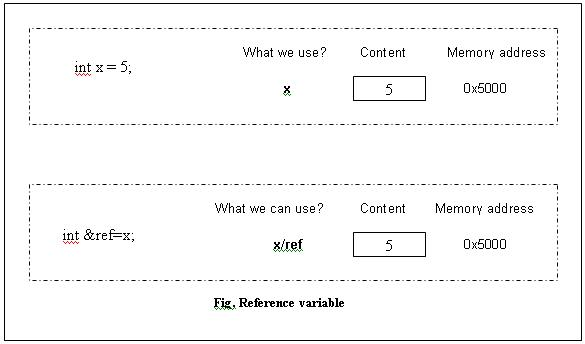More on references

• Reference variables are aliases (an alternate name to a variable).

int x;
int &ref=x;        //we tell the compiler that ‘ref’ is another name for ‘x’

Working on ‘ref’ is the same as working on ‘x’ and vice-versa.

But since a reference variable is an alias, we should initialize the alias.

int x;
int &ref;            //ILLEGAL reference variable should be initialized.

This makes sense because an alias should be an alternate name for something (and we need to tell the compiler what that something is).• Non-const references should be initialized using L-values (i.e. using something which is permitted on the left hand side of an assignment expression).

int &ref=5;       //ERROR

This is an error because ‘5’ is an integer constant and it is not an L-value (we cannot use 5 on the left side of an assignment). Logically speaking also this isn’t possible because a reference variable is an alias (you use an alias instead of another name). One might argue, “instead of the constant 5, I intended to use the term ref”. But when we think from the viewpoint of the compiler, we can understand the complications involved if this were permitted. Let’s say this statement was legal. Now (though the programmer might not purposely do it), there is nothing to stop the programmer from coding:

int &ref=5;       //ERROR (but let’s assume this is permitted)
ref=10;

What now; where is the new value of 10 supposed to be stored? (there is no location for it).

But if we use a const-reference:

const int &ref=5;          //Permitted

the compiler won’t complain. The reason is that now we tell the compiler, “This is a const reference and in case I attempt to assign any values to it then you can flag an error”. The compiler feels reassured and it will permit the use of ‘ref’ instead of 5.

Note: For the above purpose, it would be more clearer and effective to use

const int ref=5;

(since we are not really making use of any functionality of a reference variable).

• We have seen that passing by reference is similar to passing a pointer (as was done in C programming). In fact reference variables and pointers are quite similar. You might wonder, “why not use pointers itself?”

When passing a pointer, the function definition will be cluttered with a lot of dereferencing.

 Passing pointers (C style) Passing by reference void square (int *x) { *x = (*x) * (*x); } void square (int &x ) { x = x * x; }

The code is clean and reader-friendly when passing by reference.

• We can use the ‘const’ keyword in reference parameters to ensure that the function doesn’t modify the argument. For example:

//Function won’t modify the argument

void print(const int &x)
{
cout<<endl<<"The value is:"<<x;
}

void square (int &x)
{
x = x * x;
}

int main ( )
{
int y=5;
print(y);
square(y);
print(y);
return 0;

The advantage of using void print(const int &x) is that when someone reads the code later they will immediately know that the function print( ) doesn’t modify the argument.

The following:

void square (const int &x)         //compiler error
{
x = x * x;
}

is an error. The compiler will complain saying, “you are attempting to modify the value of the constant reference ‘x’”.

int x;
int &ref=x;

Both addresses will be the same (which is why working on ‘x’ is the same as working on ‘ref’).

• We can return references from functions but beware.

int& dummy( )
{
int x=5;
return x;
}

int main ( )
{
cout<<dummy( );
return 0;

The function dummy( ) is supposed to return a reference. Though the code might work as expected (an output of 5); the compiler will issue a warning (sometimes you might also get unexpected results). The warning is: “reference to local variable `x' returned”. The variable ‘x’ would be destroyed when we reach the end of the function dummy( ). A reference is just an alias. Thus when ‘x’ is destroyed, we shouldn’t use the alias of ‘x’ as well. The rule is: Don’t return references in such scenarios.

Go back to the Contents Page